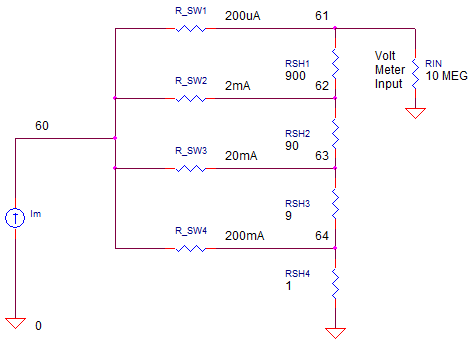eCircuit  Center DMM SERIES

### Measure DC Current

CIRCUITDigital Multi-Meters (DMMs) don't measure current directly. The idea is to insert an accurately known resistance R in series with the circuit to create a voltage proportional the current flow. Then the current can be calculated as I = V / R. This demands two competing features for the Current Sense Resistor:
1. A resistance big enough to get a decent size voltage level.
2. A resistance small enough to avoid significantly effecting the circuit's operation.

The Current Sense Resistors are also called Current Shunts. In early automobile measurements, low value resistors actually "shunted" most of the current away from analog style meters, leaving a small portion of current to drive the galvanic meter movement. Today many shunts carry all of the current, creating a voltage to be measured directly.

CURRENT SENSE RESISTOR

Similar to Decade Resistor Divider for voltage measure, the Current Sense Networks is a resistor string requiring a high level of functionality and performance.

1. Accurately create a voltage from the current flowing through it.

2. Present a LOW impedance to have a minimal effect on the circuit being measured.

3. Maintain a stable resistance with minimal change with temperature.

How does the network function? Notice the strategic placement of the switches! The problem lies in the resistance of the switches (R_SW) which could create a significant voltage. This voltage could potentially add to the measurement. The trick here is arrange the switches such that their resistance is not included in the voltage measurement. One clever solution is shown above. Let's see how it works.

For the 200uA range, only SW1 is closed. This routes the current directly to the top of sense resistor RS1. The current flows through all resistors RS1-4 for a total of .

RS = 900 + 90 + 9 + 1
= 1k

The measured voltage at V(61) is easily calculated as

Vm = RS · Im
= 1k
· 200uA
= 200 mV

For the 200mA range, only SW4 is closed and all of the current flows through RSH4 only for a resistance of  .

RS = 1 ohm

Again, the measured voltage at V(64) is calculated as

Vm = RS · Im
= 1
· 200mA
= 200 mV

NOTE!  You may have observed that in the 200 mA case, resistors RS1 through RS3 remain is series with the voltage measure point at V(61). This creates a voltage divider with RIN of the voltmeter. No problem, with RIN = 10 Meg, the voltage divider effect is insignificant!

Also note, how switch resistances R_SW0 through R_SW4 (and their associated voltage drops) are not included with the actual voltage measurement at V(61). The voltage created is related to the current sense resistors only!

RESISTOR NETWORK

If you're got a bigger budget for more accuracy, current shunts come manufactured in a single package.What are the benefits? These resistors are trimmed for accuracy and manufactured from materials to minimize the resistance change with temperature.

CURRENT MEASURE IN ACTION

Current source Im generates the current to be measured. Resistors RS1-4 form the current shunts. Switches R_SW1-4 route the input current to the appropriate shunts. These switches have all been set to OPEN (or 1e12 ohms). Finally, RIN represents the input resistance of the volt meter.

CIRCUIT INSIGHT   Choose the 200uA current scale by setting R_SW1 to 1E-1 ohms. Set Im to this 0.0002A. Run a TRANSIENT RESPONSE simulation and plot the measured voltage at V(61). Does the voltage reach 200mV for the 200uA input current?

Now change Im progressively from 200uA to 200mA in factors of 10. For each magnitude, CLOSE the appropriate switch by setting its resistance to 1E-1 and OPEN all others with a resistance of 1E12.

Rerun the TRANSIENT ANALYSIS for each magnitude of current. Does the voltage at V(61) reach 200mV max?

On the 200mA range, set R_SW4 to something large like 0.5 ohms. This is more than half of the sense resistor value! Did it have any effect on the measurement at V(61)?

DMM SERIES

Measure DC Volts with a Decade Resistor Resistor Network.
Measure DC Current with a Decade Current Sense Resistor Network.
Discover the heart of most DMM's - the Dual-Slope Integrator.

SPICE FILE

Download the file or copy this netlist into a text file with the *.cir extension.

```DMM_ADC_MEAS1.CIR
*
* INPUT VOLTAGE
IIN	0	60	0.0002A
*
* CURRENT SENSE RESISTORS
RS1	61	62	900
RS2	62	63	90
RS3	63	64	9
RS4	64	0	1
*
* SELECTOR SWITCHES
RSW_1	60 61  1e-2
RSW_2	60 62  1e12
RSW_3	60 63  1e12
RSW_4	60 64  1e12
*
* ANALYSIS *************************************************
.TRAN 	25US  1MS
.PROBE
.END```

Top ↑